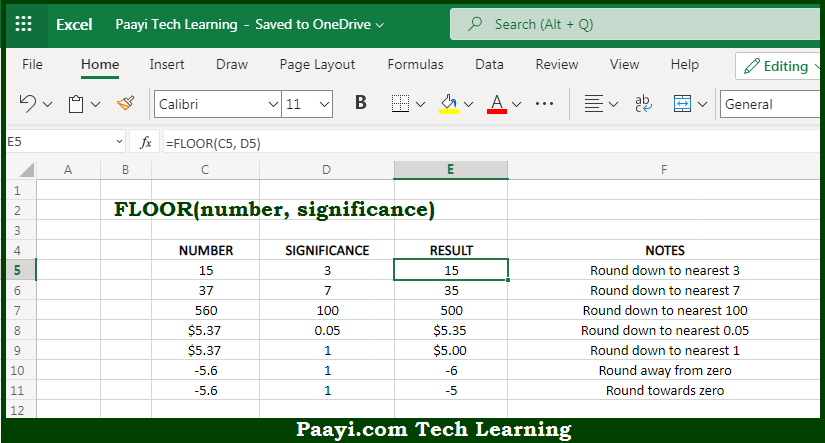# Learn How to Use Microsoft Excel FLOOR Function

Written by | 0 Comments | 566 Views

In this article, you will learn how to use the Microsoft Excel FLOOR function and its prime function in Microsoft Excel. You will also get to know the Microsoft Excel FLOOR function return value and syntax with the help of some examples.

Microsoft Excel FLOOR Function

The main function of the Microsoft Excel FLOOR function is to round down the number to the nearest specified multiple. So, with the help of the FLOOR function, you can able to round up the given number, to the nearest specified multiple. It should be noted that the FLOOR function operates similarly as the MROUND function. The only difference is that the FLOOR function always rounds down. So, with the help of the Microsoft Excel FLOOR function, you can easily round down the number to the nearest multiple.

Return Value of FLOOR Function

The return value will be the round-down number.

Syntax of FLOOR Function

=FLOOR(number, significance)

Where the arguments:

• number: This is the number of which you want to round down.
• significance: This is multiple to use while rounding down the number.

How to Use Microsoft Excel FLOOR Function?So we know that Microsoft Excel FLOOR function you can able to round down the number to the nearest specified multiple. The multiple to use for rounding is provided as the significance argument. If the number is already an exact multiple, no rounding happens and the original number is returned. So, with the help of the FLOOR function, you can able to round up the given number, to the nearest specified multiple.

It should be noted that the FLOOR function operates similarly as the MROUND function. The only difference is that the FLOOR function always rounds down. So, with the help of the Microsoft Excel FLOOR function, you can easily round down the number to the nearest multiple.